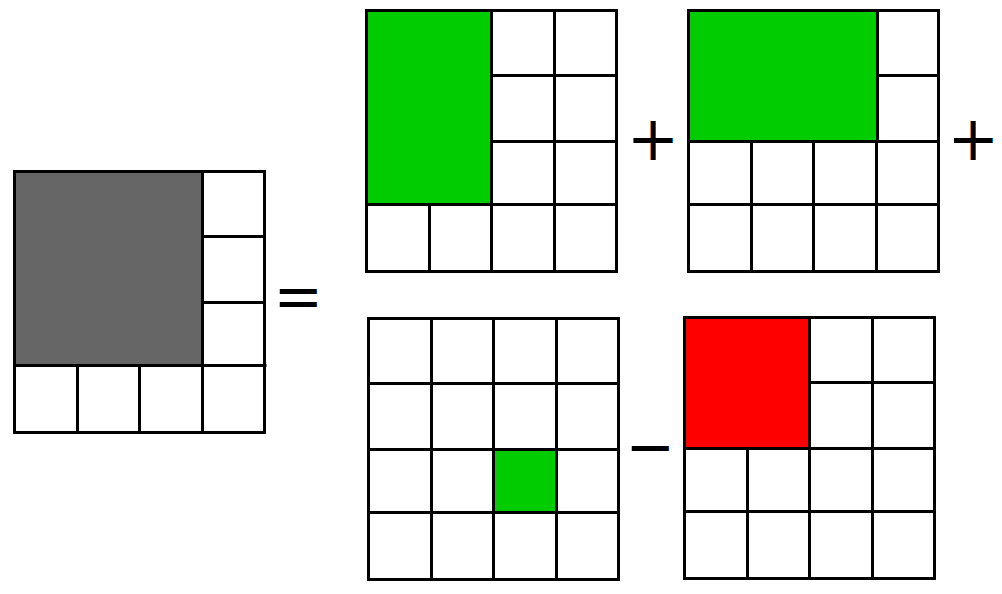# Calculate sum of all elements in a sub-matrix in constant time

Given a M x N matrix and two coordinates (p, q) and (r, s) which represents top-left and bottom-right coordinates of a sub-matrix of the given matrix, calculate the sum of all elements present in the sub-matrix in O(1) time.

Here, 0 <= p < r < M and 0 <= q < s < N

For example,

Input: matrix[][] is

[ 0 2 5 4 1 ]
[ 4 8 2 3 7 ]
[ 6 3 4 6 2 ]
[ 7 3 1 8 3 ]
[ 1 5 7 9 4 ]

(p, q) = (1, 1)
(r, s) = (3, 3)

Output:Sum is 38

Explanation:
The sub-matrix formed by coordinates (p, q), (p, s), (r, q) and (r, s) shown below having sum of elements equal to 38

[ 8 2 3 ]
[ 3 4 6 ]
[ 3 1 8 ]

The idea is to pre-process the matrix. We take an auxiliary matrix sum[][] where sum[i][j] will store the sum of the elements in matrix from (0, 0) to (i, j). We can easily calculate the value of sum[i][j] in constant time using below relation –

sum[i][j] = sum[i][j – 1] + sum[i – 1][j] + mat[i][j] – sum[i – 1][j – 1]

The following diagram easily explains this relation
(Here greyed portion represents sum of elements in the matrix from (0, 0) to (i, j))Now to calculate sum of elements present in the sub-matrix formed by coordinates (p, q), (p, s), (r, q) and (r, s) in constant time, we can directly apply below relation –

total = sum[r][s] – sum[r][q – 1] – sum[p – 1][s] + sum[p – 1][q – 1];

The following diagram explains this relation
(Here greyed portion represent the sub-matrix)Output:

38

## Java

Output:

38

Exercise:

1. Given a M x N matrix, find sum of all K x K sub-matrix

2. Given a M x N matrix and a cell (i, j), find sum of all elements of the matrix in constant time except the elements present at row i & column j of the matrix.

Related Problems: Find maximum sum K x K sub-matrix in a given M x N matrix(2 votes, average: 5.00 out of 5)Loading...

Please use our online compiler to post code in comments. To contribute, get in touch with us.
Like us? Please spread the word and help us grow. Happy coding 🙂Subscribe
Notify ofGuest
cuttlefish

These diagrams were very helpful. Thank youGuest

how is this constant time? In order to pre-process the matrix you did a 2 for loop which is O(n*m)